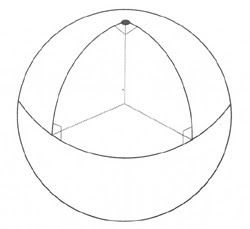## Sunday, June 21, 2015

### Triangles and Angles: a proof for 5 year olds

who: J2 (also P and J0)
what did we use: polydrons, polydron protractor

J2 and I were playing with polydrons and he got interested in measuring the various angles. Since there are three different triangles in the set, he got to test each of them and found that the angle sum was always 180 degrees. We went on to examine squares and pentagons to see what he could make of those.

In the evening, P and I were talking about about this investigation and I mentioned this proof that the interior angles of a polygon sum to $180 \times (n-2)$:

P was surprised and said that she likes this proof more:
In this version, you have to subtract the middle 360 degrees.

I was a bit surprised that I hadn't ever thought of this version or seen it elsewhere, but quickly realized we could repurpose it to finding the interior angles of a triangle itself.  Here's the picture:

Now, the argument:
1. Angles of the large triangle are equal to the sum of the angles of the smaller triangles - 360
2. Let x be the sum of interior angles of a triangle.
3. Based on 1, we have x = 3x - 360
4. Rearranging, we get x = 180.

# Where we get stuck: a challenge for you

Here's the thing: the sum of interior angle measures of a triangle isn't 180 degrees. That is, it doesn't have to be if you are working in non-euclidean geometry. Here's a nice picture (not mine) of a triangle with angle sum of 270 degrees:Comes from MathStackExchange

However, our proof doesn't seem to use any fancy postulates. Your challenge, why doesn't it work on a sphere? What extra postulate did we secretly use?

# A side point about measurement

When my son was measuring the angles for an isosceles right triangle, he read 46 degrees off the protractor. I was too focused on getting to the punchline about all triangles having the same angle sum and I "corrected" the measurement to 45 degrees. He still said some things and we had a conversation about ideal mathematics and real measurement, but I felt it was a missed opportunity.